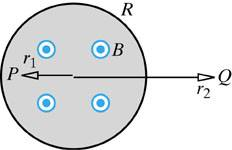# Curly Electric Fields

## Homework StatementThe magnetic field is uniform and out of the page inside a circle of radius R, and is essentially zero outside the circular region (see the figure). The magnitude of the magnetic field is changing with time; as a function of time the magnitude of the magnetic field is (B0 + bt^3). r1 = 6.4 cm, r2 = 63 cm, B0 = 1.5 T, b = 1.4 T/s3, t = 0.8 s, and R = 15 cm.

(a) What is the direction of the "curly" electric field at location P, a distance r1 to the left of the center (r1 < R)?

(b) What is the magnitude of the electric field at location P? (Hint: remember that "emf" is the integral of the non-Coulomb electric field around a closed path.)

(c) What is the direction of the "curly" electric field at location Q, a distance r2 to the right of the center (r2 > R)?

(d) What is magnitude of the "curly" electric field at location Q?

E∫dA = emf
emf=dΦB/dT
ΦB = BxA

## The Attempt at a Solution

I have no clue. It says to use the E∫dA = emf, but emf is not given. That (B0+bt^3) is just confusing me.

kuruman
Homework Helper
Gold Member
Faraday's Law says emf = -dΦ/dt. Does that help?

Not much because I don't know what to do with it. What would the flux be? Would it be
emf = -dΦ/dt

Φ = BxA

emf = (-d/dt)BxA?

Does B = B0 + bt^3?

kuruman
Homework Helper
Gold Member
Not much because I don't know what to do with it.